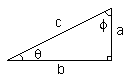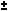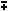Home    |    Teacher    |    Parents    |    Glossary    |    About UsEmail this page to a friendResources· Cool Tools · Formulas & Tables · References · Test Preparation · Study Tips · Wonders of MathSearchTrigonometric Identities (Math | Trig | Identities)sin(theta) = a / c csc(theta) = 1 / sin(theta) = c / a cos(theta) = b / c sec(theta) = 1 / cos(theta) = c / b tan(theta) = sin(theta) / cos(theta) = a / b cot(theta) = 1/ tan(theta) = b / a

sin(-x) = -sin(x)
csc(-x) = -csc(x)
cos(-x) = cos(x)
sec(-x) = sec(x)
tan(-x) = -tan(x)
cot(-x) = -cot(x)

 sin^2(x) + cos^2(x) = 1 tan^2(x) + 1 = sec^2(x) cot^2(x) + 1 = csc^2(x) sin(xy) = sin x cos ycos x sin y cos(xy) = cos x cosysin x sin y

tan(xy) = (tan xtan y) / (1tan x tan y)

sin(2x) = 2 sin x cos x

cos(2x) = cos^2(x) - sin^2(x) = 2 cos^2(x) - 1 = 1 - 2 sin^2(x)

tan(2x) = 2 tan(x) / (1 - tan^2(x))

sin^2(x) = 1/2 - 1/2 cos(2x)

cos^2(x) = 1/2 + 1/2 cos(2x)

sin x - sin y = 2 sin( (x - y)/2 ) cos( (x + y)/2 )

cos x - cos y = -2 sin( (x - y)/2 ) sin( (x + y)/2 )

Trig Table of Common Angles
angle 0 30 45 60 90
sin^2(a) 0/4 1/4 2/4 3/4 4/4
cos^2(a) 4/4 3/4 2/4 1/4 0/4
tan^2(a) 0/4 1/3 2/2 3/1 4/0

Given Triangle abc, with angles A,B,C; a is opposite to A, b opposite B, c opposite C:

a/sin(A) = b/sin(B) = c/sin(C) (Law of Sines)

 c^2 = a^2 + b^2 - 2ab cos(C) b^2 = a^2 + c^2 - 2ac cos(B) a^2 = b^2 + c^2 - 2bc cos(A)
(Law of Cosines)

(a - b)/(a + b) = tan [(A-B)/2] / tan [(A+B)/2] (Law of Tangents)